# Algorithm

We consider linear regression model, where we are given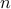i.i.d. pairs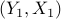,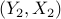,,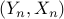with vectors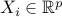and response variables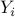are given by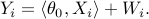Here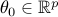is the vector of coefficients,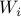is measurement noise with mean zero and variance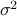. Moreover,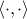denotes the standard scalar product in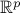.

In matrix form, letting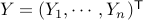and denoting by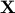the design matrix with rows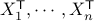, we have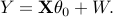Note: We are primarily interested in high-dimensional regime, where the number of parameters is larger than the number of samples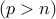, although our method applies to the classical low-dimensional setting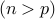as well.

Goal

Given response vector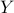and design matrix, we want to construct confidence intervals for each single coefficient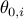.
Furthermore, we are interested in testing individual null hypothesis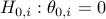versus the alternative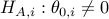, and assigning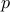-values for these tests.

Method:

Here, we provide a simple explanation of our method. For more details and discussions, please see our paper.

Our method is based on constructing a ‘de-biased’ version of LASSO.

Let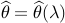be the LASSO estimator with regularization parameter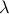. For a matrix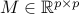, define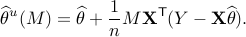For a suitable choice of matrix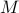, we characterize distribution of the de-biased estimator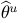, from which we construct asymptotically valid confidence intervals, as follows:

For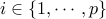and significance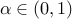, we let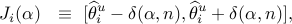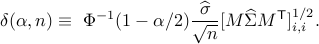Here,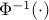is the quantile function of the standard normal distribution and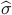is a consistent estimator of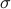.

For testing the null hypothesis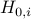, we construct a two-sided-value as follows: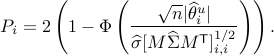How to choose matrix M?

For input parameter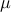, the de-biasing matrixis constructed via the following optimization problem:

1. for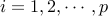do

Let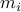be a solution of the convex program: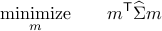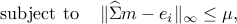where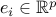is the vector with one at the-th position and zero everywhere else.

2. Set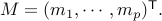( Rows ofare the vectors.)

In our code, the user can either give parametersandas input or let the algorithm select their values automatically.